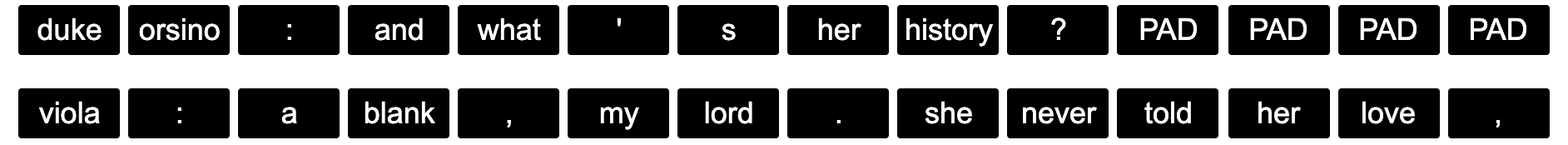### Categories

When batching inputs for sequence models you often have sequences of variable sizes and you need to pad some of the inputs so that you can input them as a single tensor. For example here is a pair of lines in a dialogue from Twelfth Night Act 2, Scene 4 which are of variable length as represented hereHowever you don’t want the pad locations to influence the weight updates. In this post we will learn how PyTorch and TensorFlow approach this via their respective embedding layers.

import torch
import tensorflow as tf
import numpy as np


Pytorch

torch.nn.Embedding(
sparse=False, _weight=None
)


Tensorflow

tf.keras.layers.Embedding(
input_dim, output_dim, embeddings_initializer='uniform',
embeddings_regularizer=None, activity_regularizer=None,
)


## padding_idx in PyTorch

From the PyTorch documentation

padding_idx (int, optional) – If specified, the entries at padding_idx do not contribute to the gradient; therefore, the embedding vector at padding_idx is not updated during training, i.e. it remains as a fixed “pad”. For a newly constructed Embedding, the embedding vector at padding_idx will default to all zeros, but can be updated to another value to be used as the padding vector.

embed_size = 7
lin_pyt = torch.nn.Linear(1, 1)
arr = np.stack([[1, 1, 2, 6, 0],
[1, 5, 5, 0, 0]])
inp_pyt = torch.from_numpy(arr)


Run forward pass weighting each location randomly so to force the gradients for each embedding to be different:

z = lin_pyt(embed_pyt(inp_pyt))
weight = torch.rand_like(z)
z2 = torch.sum(z*weight)
z2.backward()


Note that the weight for padding_idx i.e. 0 here is zero

embed_pyt.weight

Parameter containing:
tensor([[ 0.0000],
[-0.1074],
[ 0.6048],
[ 1.1213],
[-0.6248],
[ 0.3374],


The grad values are typically larger for the tokens that occur more often (1 and 5 v 2 and 6) and zero for those that don’t appear (3, 4) and 0 for padding_idx=0.

embed_pyt.weight.grad

tensor([[0.0000],
[0.0380],
[0.0080],
[0.0000],
[0.0000],
[0.0398],
[0.0347]])


## mask_zero in TensorFlow

This parameter serves a similar purpose to padding_idx above

mask_zero: Boolean, whether or not the input value 0 is a special “padding” value that should be masked out. This is useful when using recurrent layers which may take variable length input. If this is True, then all subsequent layers in the model need to support masking or an exception will be raised. If mask_zero is set to True, as a consequence, index 0 cannot be used in the vocabulary (input_dim should equal size of vocabulary + 1).

However it works differently. It won’t affect the output of the embedding layer. Instead it will return a tensor that can be used for blocking the pad positions in subsequent layers.

First let us construct a similar model in TensorFlow reusing the input from before

embed_tf = tf.keras.layers.Embedding(embed_size, 1, mask_zero=True)
inp_tf = tf.convert_to_tensor(arr)


Now we will create simpled masked wrapper for Dense the equivalent of Linear in PyTorch.

class MaskedDense(tf.keras.layers.Dense):

tf.constant_initializer(lin_pyt.weight.detach().numpy()))


Now get the outputs and gradients

with tf.GradientTape() as tape:
z = lin_tf(embed_tf(inp_tf))
z2 = tf.reduce_sum(z * weight.numpy())



Note that the weights here are not zero for the padding index of 0.

embed_tf.weights

<tf.Variable 'embedding/embeddings:0' shape=(7, 1) dtype=float32, numpy=
array([[ 0.01041734],
[ 0.04714436],
[ 0.04238981],
[ 0.01394412],
[ 0.00850029],
[-0.0347899 ],
[ 0.00357641]], dtype=float32)>


grad

<tensorflow.python.framework.indexed_slices.IndexedSlices at 0x7fd851f25dc0>


The gradient for Embedding is an instance of IndexedSlices so we need to reconstruct tensor from it.

tf.scatter_nd(grad.indices[:, None], grad.values, tf.cast(grad.dense_shape, tf.int64))

<tf.Tensor: shape=(7, 1), dtype=float32, numpy=
array([[0.        ],
[0.03796878],
[0.00800363],
[0.        ],
[0.        ],
[0.03977239],
[0.03467343]], dtype=float32)>


As expected the gradient for 0 does have a value of zero.